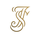1391 views
So my goal here was to combine all of my RSI ideas into a single indicator in order to make kind of a "Swiss Army Knife" version of the Relative Strength Index ...

------------------------------------------------------------------------------------------------------------------------------------------------------------------------------------------------------------------------------------------------------------------

So, let's begin with the first RSI indicator I made, which is the RSIDVW (Divergence/Volume Weighted);

To rephrase my original post, the "divergence/volume weighted" portion is meant to expand upon the current RSI format by adding more variables into the equation.
The standard RSI is based off one value that you select (open, close, OHLC4, HLC3, etc.) while this version takes three variables into account.

The default setting is to have RSI normal without anything added to it (Divergence Weight = 0)

1st - it takes the standard variable that RSI normally uses.
2nd - it factors RSI divergence by taking the RSI change % and price change % to form a ratio. Using this ratio, I duplicated the RSI formula and created a divergence RS to be factored in with the standard price RS .
3rd - it takes Relative Volume and amplifies/weakens the move based upon volume confirmation. (So if Relative Volume for a price bar is 1.0, the RSI plot would be the same as it normally would)

So to explain the parameters
- Relative Volume Length: This uses the RV length you specify to determine spikes in volume (or lack of volume ), which then is added into the formula to influence the strength of the RSI move
- RV x Divergence: This is how I calculated the original formula, but you can leave this unchecked to turn Relative Volume off, or apply elsewhere.
- RV x RS: There's two sides, Divergence RS and Standard RS - these check marks allow you to select which part you prefer to be multiplied by Relative Volume .

Checking neither turns off Relative Volume , while checking both amplifies its effects by placing it on both sides of the equation.

-Divergence Weight: This controls how much the DVW portion of the formula influences the RSI plot. As I referred to earlier, default is 0 making RSI normal. The Scale is 0-2, so 1.0 would be the same as 50%.

When I do have DVW on, I generally set it to 0.5

-SMA Divergence: To smooth, or not to smooth, that is the question. UJsing an SMA here is much smoother in my opinon, but leaving it unchecked runs it through an RMA the same way standard RSI is calculated.
-Show Fractal Channel: This allows you to see the whole fractal channel around the RSI (This portion of the code, compliments of the original Ricardo Santos fractal script)

------------------------------------------------------------------------------------------------------------------------------------------------------------------------------------------------------------------------------------------------------------------

The next portion of the script is adding a "Slow RSI"...

This is rather simple really, it allows you to add a second RSI plot so that you can watch for crossovers between fast and slow lines.

-Slow RSI: This turns on the second RSI Plot.
-Slow RSI Length: This determines the length of the second RSI Plot.

------------------------------------------------------------------------------------------------------------------------------------------------------------------------------------------------------------------------------------------------------------------

Pivot Point RSI was something a friend of mine requested I make which turned out pretty cool, I thought... It is also available in this indicator.

-Pivot Points: Selecting this enables the rest of the pivot point related parts of the script

If Pivot Points isn't selected, none of the following things will work

-Plot Pivot: Plots the pivot point .
-Plot S1/R1: Plots S1/R1.
-Plot S2/R2: Plots S2/R2.
-Plot S3/R3: Plots S3/R3.
-Plot S4/R4: Plots S4/R4.
-Plot S5/R5: Plots S5/R5.
-Plot Halfway Points: Plots a line between each pivot .
-Show Pivot Labels: Shows the proper label for each pivot .

When using intraday charts, from a 15 minute interval or less the pivots are calculated based on a single days worth of price action, above that the distance expands.

Here are the current resolutions Pivot Points will work with:
Minutes - 1 , 2, 3, 5, 10, 13, 15, 20, 30, 39, 78, 130, 195
Hours - 1, 2, 3, 4, 5, 6
Daily
Weekly

Currently not available on seconds or monthly

------------------------------------------------------------------------------------------------------------------------------------------------------------------------------------------------------------------------------------------------------------------

Background Colors

Background Colors: I have six color schemes I created for this which can be toggled here (they can be edited).
Gray Background for Dark Mode: Having this on looks much better when using dark mode on your charts.

------------------------------------------------------------------------------------------------------------------------------------------------------------------------------------------------------------------------------------------------------------------

Now finally the last portion, Fibonacci Levels

-Fibonacci Levels: This is off, by default, which then uses the standard levels on RSI (30-50-70). When turned on, it removes these and marks fib levels from 0.146 through 0.886.

------------------------------------------------------------------------------------------------------------------------------------------------------------------------------------------------------------------------------------------------------------------

So the quick rundown:

Ultimate RSI contains "divergence/volume weighted" modifications, a slow RSI plot, pivot points , and Fibonacci levels all while auto-plotting divergence and having the trend illustrated in the background colors.

RSI has always been my "go to" indicator, so I hope you all enjoy this as much as I do!

Versionshinweise: Changed the default values so nothing has to be changed on charts that don't contain volume.
Versionshinweise: Added the ability to chart the monthly, and all other time frames not covered by the Pivot Point portion of the code.

## KommentareThis is a very sophisticated version of a very simple indicator. I like it, good job. Definitely will explore it and give further feedback after using it for a longer period.

When you have your indicators enabled, it shows the current value based on what the indicator is doing. For example, the RSI is 51.33 and the slow RSI is 45.29 and it shows those on the chart. I would like to know how to remove all the n/a outputs that are next to the RSI and slow RSI display. No idea where they are coming from, please help :)
Antwortenkrvpto
@krvpto, the N/A's are the pivot points I believe, you'd have to remove that portion of coding
Antworten